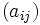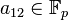Center of unitriangular matrix group:UT(3,p)

This article is about a particular subgroup in a group parametrized by a prime (so we get one group for each prime and a corresponding subgroup for it), up to equivalence of subgroups (i.e., an isomorphism of groups that induces the corresponding isomorphism of subgroups). The subgroup is (generically) group of prime order and the group is (generically) prime-cube order group:U(3,p) (see subgroup structure of prime-cube order group:U(3,p)).
The subgroup is a normal subgroup and the quotient group is (generically) elementary abelian group of prime-square order.
VIEW: Group-subgroup pairs with the same subgroup part | Group-subgroup pairs with the same group part| Group-subgroup pairs with the same quotient part | All pages on prime-parametrized particular subgroups in groups

Definition

Let$p$ be an odd prime (The case$p = 2$, where we get center of dihedral group:D8, is somewhat different, though many things are similar).

The group$G = U(3,p)$ is the group of unipotent upper-triangular matrices over the prime field of$p$ elements, and is also the unique (up to isomorphism) non-abelian group of order$p^3$ and exponent$p$. It is defined by the presentation:$G := \langle g,h,k \mid g^p = h^p = k^p = e, gh = hg, gk = kg, khk^{-1} = g^{-1}h \rangle$.

In the matrix description, each matrix$(a_{ij})$ can be described by the three entries$a_{12}, a_{13}, a_{23}$. The matrix looks like:$\begin{pmatrix} 1 & a_{12} & a_{13} \\ 0 & 1 & a_{23}\\ 0 & 0 & 1\end{pmatrix}$

The multiplication of matrices$A = (a_{ij})$ and$B = (b_{ij})$ gives the matrix$C = (c_{ij})$ where:

•$c_{12} = a_{12} + b_{12}$
•$c_{13} = a_{13} + b_{13} + a_{12}b_{23}$
•$c_{23} = a_{23} + b_{23}$

With the matrix description, we can set$g$ as the matrix with$a_{13} = 1$ and the other two entries zero,$h$ as the matrix with$a_{12} = 1$ and the other two entries zero, and$k$ as the matrix with$a_{23} = 1$ and the other two entries zero.

Now define the subgroup:$H := \{ \begin{pmatrix} 1 & 0 & a_{13} \\ 0 & 1 & 0\\ 0 & 0 & 1\end{pmatrix} : a_{13} \in \mathbb{F}_p \}$

In other words, this is the subgroup given by$a_{12} = a_{23} = 0$.

Cosets

The subgroup has$p^2$ cosets each of size$p$, where each coset is parametrized by fixed values of$a_{12} and [itex]a_{23}$. In other words, for any fixed$a_{12} \in \mathbb{F}_p$ and$a_{23} \in \mathbb{F}_p$, the corresponding coset of$H$ in$G$ is the set of all matrices with those values of$a_{12}$ and$a_{23}$, and with$a_{13}$ varying over all of$\mathbb{F}_p$.

Complements

The subgroup has no permutable complements and also no lattice complements.

Properties related to complementation

Property Meaning Satisfied? Explanation
complemented normal subgroup normal subgroup with a complement No nilpotent and non-abelian implies center is not complemented
permutably complemented subgroup subgroup with a permutable complement. No nilpotent and non-abelian implies center is not complemented
lattice-complemented subgroup subgroup with a lattice complement. No nilpotent and non-abelian implies center is not complemented

Effect of subgroup operators

Function Value as subgroup (descriptive) Value as subgroup (link) Value as group
normalizer the whole group -- prime-cube order group:U(3,p)
centralizer the whole group current page prime-cube order group:U(3,p)
normal core the subgroup itself current page group of prime order
normal closure the subgroup itself current page group of prime order
characteristic core the subgroup itself current page group of prime order
characteristic closure the subgroup itself current page group of prime order
commutator with whole group the trivial subgroup current page trivial group

Subgroup-defining functions

The subgroup is a characteristic subgroup of the whole group and arises as a result of many subgroup-defining functions on the whole group. Some of these are given below.

Subgroup-defining function Meaning in general Why it takes this value
center set of elements that commute with every element To see that every element of$H$ is in the center, simply use the formula for multiplication and simplify algebraically. The same algebraic approach can be used to show that nothing else is in the center.
derived subgroup subgroup generated by commutators of all pairs of elements in the group, smallest subgroup with abelian quotient The quotient (called the abelianization) is$G/H$, which is isomorphic to elementary abelian group of prime-square order. No smaller subgroup works, because the quotient by the trivial subgroup is isomorphic to$G$, which is non-abelian.
socle subgroup generated by all the minimal normal subgroups It is the unique minimal normal subgroup (hence is also a monolith). In general, for a finite$p$-group, the socle is$\mho^1(Z(G))$.
Frattini subgroup intersection of all the maximal subgroups$H$ is the intersection of maximal subgroups obtained by taking$H$ along with any single element outside it.
ZJ-subgroup center of the join of abelian subgroups of maximum order The join of abelian subgroups of maximum order (the Thompson subgroup) is the whole group, so its center is$H$.

Characteristicity and related properties satisfied

Property Meaning Satisfied? Explanation
normal subgroup invariant under inner automorphisms Yes center is normal
characteristic subgroup invariant under all automorphisms Yes center is characteristic, derived subgroup is characteristic
fully invariant subgroup invariant under all endomorphisms Yes derived subgroup is fully invariant
verbal subgroup generated by set of words Yes derived subgroup is verbal
normal-isomorph-free subgroup no other isomorphic normal subgroup Yes
isomorph-free subgroup, isomorph-containing subgroup No other isomorphic subgroups No There are other subgroups of order$p$. In fact, every element of the group has order$p$.
isomorph-normal subgroup Every isomorphic subgroup is normal No There are other subgroups of order two that are not normal:$\langle x \rangle$ etc.
homomorph-containing subgroup contains all homomorphic images No There are other subgroups of order$p$.